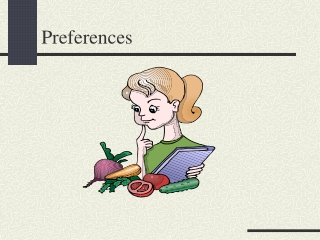DownloadDownload PresentationPreferences

# Preferences

Download Presentation## Preferences

- - - - - - - - - - - - - - - - - - - - - - - - - - - E N D - - - - - - - - - - - - - - - - - - - - - - - - - - -
##### Presentation Transcript

1. Preferences

2. Preference Relation • The consumer strictly prefers bundle X to bundle Y: • The consumer is indifferent between X and Y:

3. Weak Preference • If or • Then:

4. How are the relations related? Q: What do these two relations imply?

5. How are the relations related? A: The consumer is indifferent between X and Y:

6. Assumption I: Complete Preferences For any two bundles X and Y: • X preferred to Y: • Y preferred to X: • Indifference:

7. Assumption II: Reflexive Any bundle X is at least as good as itself:

8. Assumption III: Transitive • If: • And: • Then:

9. Indifference curves Indifference curve Weakly preferred set

10. Q: Can indifference curves cross?

11. Perfect substitutes

12. Perfect complements

13. Satiation

14. Well-behaved preferences • Let’s impose some extra assumptions to rule out less interesting situations • Well-behaved preferences satisfy two properties: • Monotonicity • Convexity

15. Monotonicity • Consider two bundles: where Y has at least as much of both goods and more of one. • Then: • Monotonicity implies that indifference curves have negative slopes

16. Indifference curves have negative slopes

17. Convexity • Consider two bundles: • Convexity implies that, for

18. Convex preferences

19. Non-convex preferences

20. The MRS is the slope of the indifference curve at a point MRS=derivative of indifference curve Marginal rate of substitution

21. Interpretation of MRS • The MRS measures the rate at which the consumer is willing to substitute one good for the other. • If good 2 is measured in dollars, the MRS measures the consumer’s willingness to pay for an extra unit of good 1.